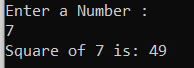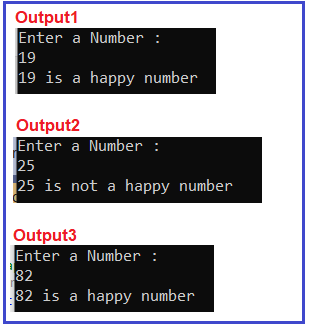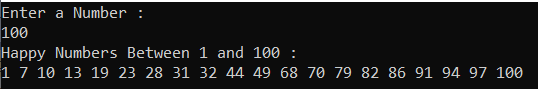# Happy Number in C#

## Happy Number Program in C# with Examples

In this article, I am going to discuss the Happy Number Program in C# with Examples. Please read our previous article where we discussed the Vampire Number Program in C#. Here, in this article, first, we will learn what is a Happy Number and then we will see how to implement the Happy Number Program in C#. And finally, we will see how to print all the Happy Numbers between a range of numbers like between 1 to 100 or 100 to 1000, etc.

##### What is a Happy Number?

A happy number is a number that reaches 1 after a sequence of steps. In each step, the number is replaced by the sum of its squared digits.  Or

A number is called happy if it leads to 1 after a sequence of steps wherein each step number is replaced by the sum of squares of its digit that is if we start with Happy Number and keep replacing it with digits square sum, we reach 1.

Example1:
Input: n = 19
Output: True
Explanation:
12 + 92 = 1 + 81 = 82
82 + 22 = 64 + 4 = 68
62 + 82 = 36 + 64 = 100
12 + 02 + 02 = 1 + 0 + 0 = 1
As we reached to 1, so, 19 is a Happy Number.’

Example2:
Input: n = 20
Output: False
Explanation:
22 + 02 = 4 + 0 = 4, So 20 is not a happy number

First of all, we have to know what is Square number.

##### SQUARE NUMBER:

A square number is the result when a number has been multiplied by itself. For example, 25 is a square number because it’s 5 x 5.

##### Square Number Program in C#:

The following program shows the Square Number Program in C#.

```using System;
public class SquareNumber
{
public static void Main()
{
Console.WriteLine("Enter a Number : ");
double Sqr = Math.Pow(Number, 2);
Console.WriteLine("Square of {0} is: {1}", Number, Sqr);
}
}
```
###### Output:##### How to Implement Happy Number Program in C#?

In order to implement the Happy Number Program in C#, we are going to use some operators and methods. We are going to use the very basic method to implement this program but before that, you should have some basic knowledge of the following operators.

1) Modulus %: Modulus Operator will calculate the remainder after an integer division.
Example: 82 % 10 = 2
68 % 10 = 8

2) Divides /: Divides numerator by de-numerator
Example: 82/ 10 = 8
68/ 10 = 6

##### Algorithm to Implement Happy Number:

The idea is very simple, calculate the square of each digit present in the number and add it to a variable sum. If the resulting sum is equal to 1 then the given number is a happy number. Otherwise, it is not a happy number.

###### Here are the steps to follow: –

1) IsHappyNumber() determines whether a given number is a happy number or not.

1. If the number is greater than 0, then calculate remainder rem by dividing the number by 10.
2. Calculate the square of rem and add it to a variable sum.
3. Divide number by 10.
4. Repeat the steps from a to c till the sum of the square of all digits present in the number has been calculated.
5. Finally, return the sum.

2) Define and initialize variable num.
3) Define a variable result and initialize it with a value of num.
4) If the result is neither equal to 1 nor 4 then, make a call to IsHappyNumber().
5) Otherwise, if the result is equal to 1 then, the given number is a happy number.
6) If the result is equal to 4 then, the given number is not a happy number.

##### Example: Happy Number Program in C#

The following code shows how to implement the Happy Number program in C#.

```using System;
public class HappyNumber
{
//IsHappyNumber() will determine whether a number is happy or not
public static int IsHappyNumber(int num)
{
int rem = 0, sum = 0;
//Calculates the sum of squares of digits
while (num > 0)
{
rem = num % 10;
sum = sum + (rem * rem);
num = num / 10;
}
return sum;
}

public static void Main()
{
Console.WriteLine("Enter a Number : ");
int result = Number;
while (result != 1 && result != 4)
{
result = IsHappyNumber(result);
}

//Happy number always ends with 1
if (result == 1)
Console.WriteLine(Number + " is a happy number");

//Unhappy number ends in a cycle of repeating numbers which contains 4
else if (result == 4)
Console.WriteLine(Number + " is not a happy number");

}
}
```
###### Output:##### C# Program to Print all Happy Numbers Between 1 and N

The following C# Program will allow the user to input a number and then it will print all the Happy numbers between 1 and that input number.

```using System;
public class HappyNumber
{
//IsHappyNumber() will determine whether a number is happy or not
public static int IsHappyNumber(int num)
{
int rem = 0, sum = 0;
//Calculates the sum of squares of digits
while (num > 0)
{
rem = num % 10;
sum = sum + (rem * rem);
num = num / 10;
}
return sum;
}

public static void Main()
{
Console.WriteLine("Enter a Number : ");
Console.WriteLine("Happy Numbers Between 1 and " + Number + " : ");

for (int i = 1; i<= Number; i++)
{
int num = i;
int result = num;
while (result != 1 && result != 4)
{
result = IsHappyNumber(result);
}

//Happy number always ends with 1
if (result == 1)
{
Console.Write(num + " ");
}
}